# Using the convdistr package

#### 2021-04-19

The convdistr package provide tools to define distribution objects and make mathematical operations with them. It keep track of the results as if they where scalar numbers but maintaining the ability to obtain randoms samples of the convoluted distributions.

To install this package from github

devtools::install_github("johnaponte/convdistr", build_manual = T, build_vignettes = T)

## Practical example

What would be the resulting distribution of $$a+b*c$$ if $$a$$ is a normal distribution with mean 1 and standard deviation 0.5, $$b$$ is a poisson distribution with lambda 5 and $$c$$ is a beta distribution with shape parameters 10 and 20?

library(convdistr)
library(ggplot2)

a <- new_NORMAL(1,0.5)
b <- new_POISSON(5)
c <- new_BETA(10,20)
res <- a + b * c

#>   distribution     rvar
#> 1  CONVOLUTION 2.666667
summary(res)
distribution varname oval nsample mean_ sd_ lci_ median_ uci_
CONVOLUTION rvar 2.67 10000 2.67 1.02 0.92 2.59 4.94
ggDISTRIBUTION(res) + ggtitle("a + b * c")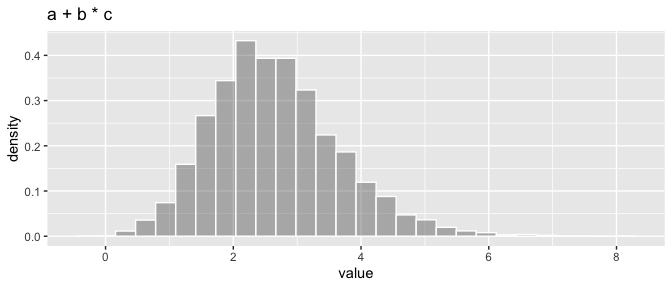The result is a distribution with expected value 2.67. A sample from 10000 drawns of the distribution shows a mean value of 2.67, a median of 2.59 and 95% quantiles of 0.92, 4.94

The following sections describe the DISTRIBUTION object, how to create new DISTRIBUTION objects and how to make operations and mixtures with them.

Please note that when convoluting distributions, this package assumes the distributions are independent between them, i.e. their correlation is 0. If not, you need to implement specific distributions to handle the correlation, like the MULTIVARIATE object.

## Description of the DISTRIBUTION object

The DISTRIBUTION is kind of abstract class (or interface) that specific constructors should implement.

It contains 4 fields:

distribution : A character with the name of the distribution implemented

seed : A numerical seed that is use to get a repeatable sample in the summary function

oval : The observed value. It is the value expected. It is used as a number for the mathematical operations of the distributions as if they were a simple scalar

rfunc(n) : A function that generate random numbers from the distribution. Its only parameter n is the number of drawns of the distribution. It returns a matrix with as many rows as n, and as many columns as the dimensions of the distributions

The DISTRIBUTION object can support multidimensional distributions for example a dirichlet distribution. The names of the dimensions should coincides with the names of the oval vector. If it has only one dimension, the default name is rvar.

It is expected that the rfunc could be included in the creation of new distributions by convolution or mixture, so the environment should be carefully controlled to avoid reference leaking that is possible within the R language. For that reason, the rfunc should be created within a restrict_environment function that controls that only the variables that are required within the function are saved in the environment of the function.

Once the new objects are instanced, the fields are immutable and should not be changed.

## Factory of DISTRIBUTION objects

The following functions create new objects of class DISTRIBUTION

Distribution factory parameters function
uniform new_UNIFORM p_min, p_max runif
normal new_NORMAL p_mean, p_sd rnorm
beta new_BETA p_shape1, p_shape2 rbeta
beta new_BETA_lci p_mean, p_lci, p_uci rbeta
triangular new_TRIANGULAR p_min, p_max, p_mode rtriangular
poisson new_POISSON p_lambda rpoisson
exponential new_EXPONENTIAL p_rate rexp
discrete new_DISCRETE p_supp, p_prob sample
dirichlet new_DIRICHLET p_alpha, p_dimnames rdirichlet
truncated new_TRUNCATED p_distribution, p_min, p_max
dirac new_DIRAC p_value
NA new_NA p_dimnames

## Methods

The following are methods for all objects of class DISTRIBUTION

• metadata(x) Print the metadata for the distribution
• summary(object, n=10000) Produce a summary of the distribution
• rfunc(x, n) Generate n random drawns of the distribution
• plot(x, n= 10000) Produce a density plot of the distribution
• ggDISTRIBUTION(x, n= 10000) produce a density plot of the distribution using ggplot2
myDistr <- new_NORMAL(0,1)
#>   distribution rvar
#> 1       NORMAL    0
rfunc(myDistr, 10)
#>          rvar
#> 1  -0.4001667
#> 2  -0.5890651
#> 3  -2.1808270
#> 4   0.1727349
#> 5   0.0114702
#> 6   0.6959998
#> 7  -0.8604288
#> 8  -0.8342938
#> 9   0.4596868
#> 10  0.5007938
summary(myDistr)
distribution varname oval nsample mean_ sd_ lci_ median_ uci_
NORMAL rvar 0 10000 0 1 -1.97 0 1.94
plot(myDistr)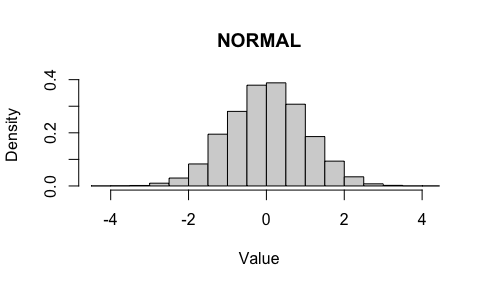Figure with R plot

ggDISTRIBUTION(myDistr)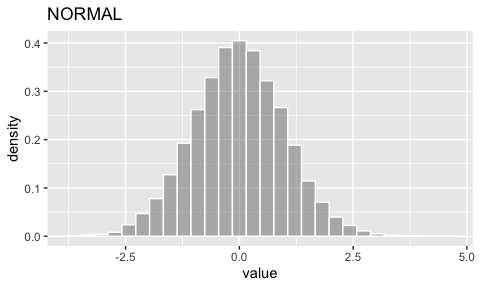Figure with ggplot2

## Convolution for Distribution with the same dimensions

Mathematical operations like +, -, *, / between DISTRIBUTION with the same dimensions can be perform with the new_CONVOLUTION(listdistr, op, omit_NA = FALSE) function. The listdistr parameter is a list of DISTRIBUTION objects on which the operation is made. A shorter version exists for each one of the operations as follow

• new_SUM(listdistr, omit_NA = FALSE)
• new_SUBTRACTION(listdistr, omit_NA = FALSE)
• new_MULTIPLICATION(listdistr, omit_NA = FALSE)
• new_DIVISION(listdistr, omit_NA = FALSE)

but Mathematical operator can also be used.

d1 <- new_NORMAL(1,1)
d2 <- new_UNIFORM(2,8)
d3 <- new_POISSON(5)
dsum <- new_SUM(list(d1,d2,d3))
dsum
#>   distribution rvar
#> 1  CONVOLUTION   11
d1 + d2 + d3
#>   distribution rvar
#> 1  CONVOLUTION   11
summary(dsum)
distribution varname oval nsample mean_ sd_ lci_ median_ uci_
CONVOLUTION rvar 11 10000 11.03 2.98 5.53 10.96 17.14
ggDISTRIBUTION(dsum)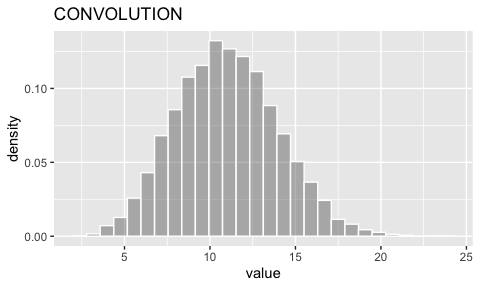## Mixture

A DISTRIBUTION, consisting on the mixture of several distribution can be obtained with the new_MIXTURE(listdistr, mixture) function where listdistr is a list of DISTRIBUTION objects and mixture the vector of probabilities for each distribution. If missing the mixture, the probability will be the same for each distribution.

d1 <- new_NORMAL(1,0.5)
d2 <- new_NORMAL(5,0.5)
d3 <- new_NORMAL(10,0.5)
dmix <- new_MIXTURE(list(d1,d2,d3))
summary(dmix)
distribution varname oval nsample mean_ sd_ lci_ median_ uci_
MIXTURE rvar 5.33 10000 5.35 3.73 0.31 5 10.73
ggDISTRIBUTION(dmix)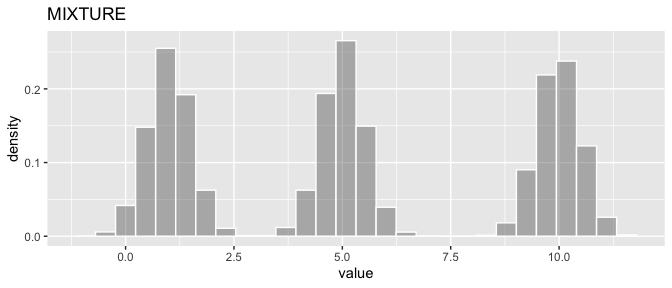## Convolution of distributions with different dimensions

When convoluting distribution with different dimensions, there are two possibilities. The new_CONVOLUTION_assoc family of functions perform the operation only on the common dimensions and left the others dimensions as they are, or the new_CONVOLUTION_comb family of functions which perform the operation in the combination of all dimensions.

d1 <- new_MULTINORMAL(c(0,1), matrix(c(1,0.3,0.3,1), ncol = 2), p_dimnames = c("A","B"))
d2 <- new_MULTINORMAL(c(3,4), matrix(c(1,0.3,0.3,1), ncol = 2), p_dimnames = c("B","C"))
summary(d1)
distribution varname oval nsample mean_ sd_ lci_ median_ uci_
MULTINORMAL A 0 10000 0.00 1 -1.93 0.01 1.96
MULTINORMAL B 1 10000 1.01 1 -0.95 1.01 2.92
summary(d2)
distribution varname oval nsample mean_ sd_ lci_ median_ uci_
MULTINORMAL B 3 10000 3 0.99 1.07 3.01 4.92
MULTINORMAL C 4 10000 4 1.00 1.98 4.01 5.99
summary(new_SUM_assoc(d1,d2))
distribution varname oval nsample mean_ sd_ lci_ median_ uci_
CONVOLUTION A 0 10000 -0.01 1.01 -1.97 -0.02 1.98
CONVOLUTION C 4 10000 4.00 1.01 2.06 3.99 6.00
CONVOLUTION B 4 10000 4.00 1.41 1.23 4.01 6.77
summary(new_SUM_comb(d1,d2))
distribution varname oval nsample mean_ sd_ lci_ median_ uci_
CONVOLUTION A_B 3 10000 3.00 1.43 0.20 3.01 5.84
CONVOLUTION B_B 4 10000 3.99 1.42 1.21 3.99 6.79
CONVOLUTION A_C 4 10000 4.01 1.42 1.22 3.99 6.81
CONVOLUTION B_C 5 10000 4.99 1.40 2.24 5.00 7.74